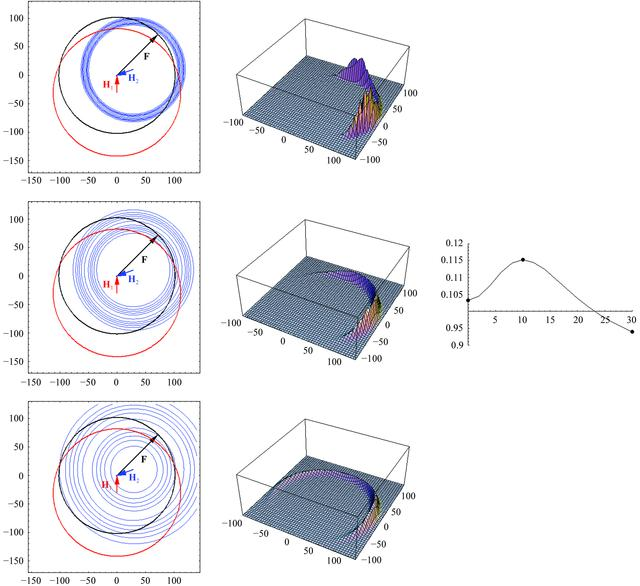disable zoom     view article Figure 4 Representation of likelihood as a function of assumed lack-of-isomorphism error for one derivative. The panels on the left show probability distributions for three possible choices of lack-of-isomorphism error for the derivative, which changes the width of the distributions. The combined distribution from the native and two derivative amplitudes (multiplied by the prior probability of the true structure factor, as in equation 7) is shown as a surface plot in the three middle panels. The likelihood is the volume under this surface and the panel on the right shows the likelihood as a function of the lack-of-isomorphism error, with dots highlighting the values corresponding to the three pairs of plots to the left. Likelihood is maximized when the distribution is just broad enough to overlap with the other distributions. An animated version of this figure is available as supplementary material1.BIOLOGICALCRYSTALLOGRAPHY
ISSN: 1399-0047
Volume 59| Part 11| October 2003| Pages 1891-1902